COMMON CORE STANDARDS COLLEGE AND CAREERREADINESS STANDARDS FLORIDAS

• Slides: 30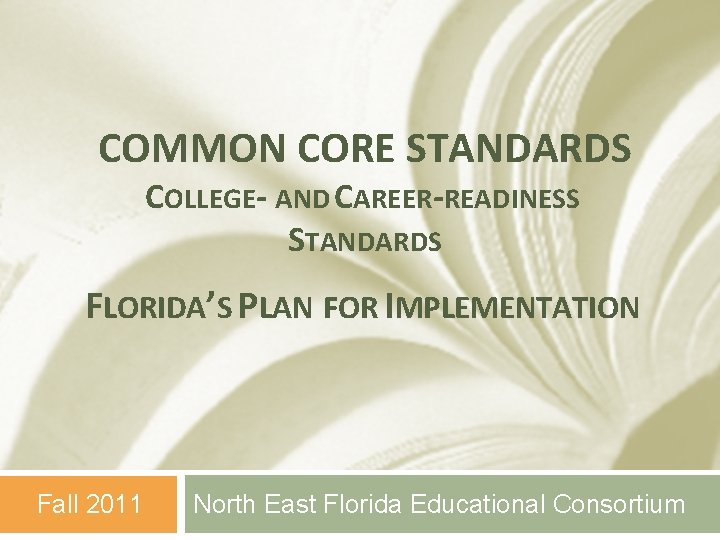COMMON CORE STANDARDS COLLEGE- AND CAREER-READINESS STANDARDS FLORIDA’S PLAN FOR IMPLEMENTATION Fall 2011 North East Florida Educational ConsortiumLearning Goals q Teachers will know the design and organization of the Mathematics Common Core Standards. q Teachers will understand that the Mathematical Practices and Mathematic Content Standards describe varieties of expertise that mathematics educators should seek to develop in their students. q Teachers will be able to integrate the Mathematical Practices and Mathematic Content Standards when developing student learning goals. Your Personal Goal Essential Question: How can you develop learning goals for your students using the Common Core Mathematic 2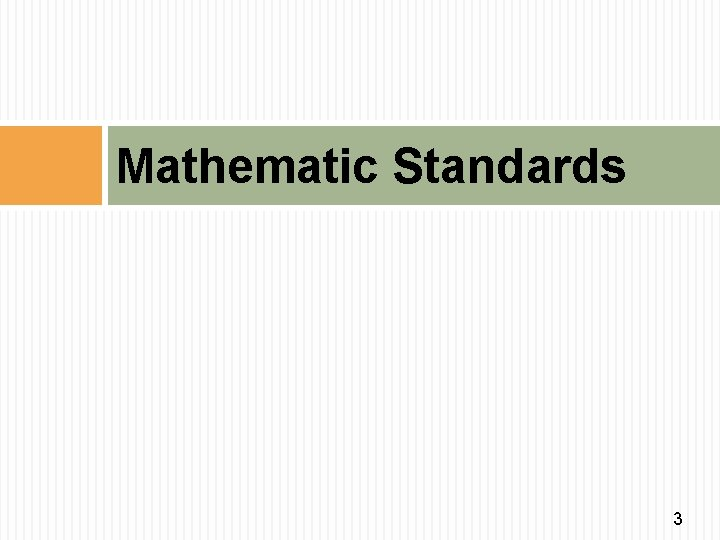Mathematic Standards 3Mathematical Practices v The Common Core proposes a set of Mathematical Practices that all teachers should develop in their students. v These practices are similar to NCTM’s Mathematical Processes from the Principles and Standards for School Mathematics. 4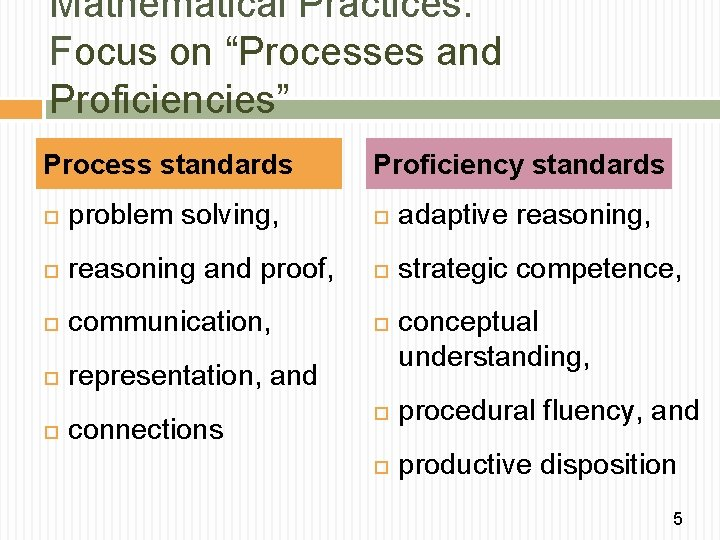Mathematical Practices: Focus on “Processes and Proficiencies” Process standards Proficiency standards problem solving, adaptive reasoning, reasoning and proof, strategic competence, communication, representation, and connections conceptual understanding, procedural fluency, and productive disposition 5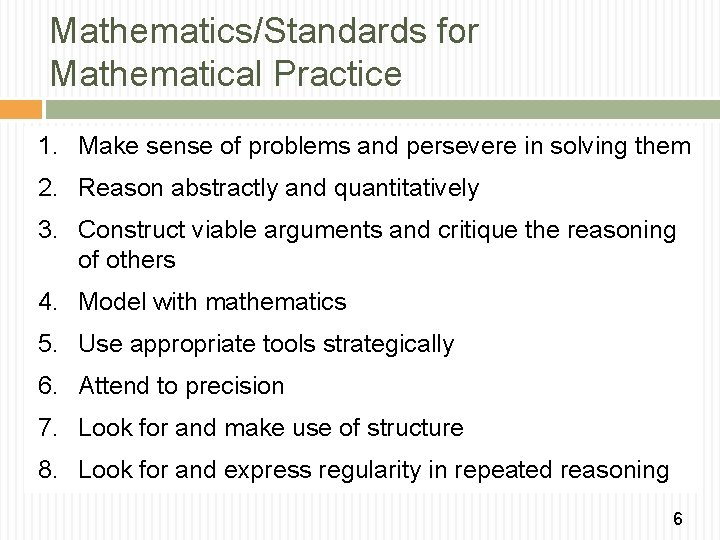Mathematics/Standards for Mathematical Practice 1. Make sense of problems and persevere in solving them 2. Reason abstractly and quantitatively 3. Construct viable arguments and critique the reasoning of others 4. Model with mathematics 5. Use appropriate tools strategically 6. Attend to precision 7. Look for and make use of structure 8. Look for and express regularity in repeated reasoning 6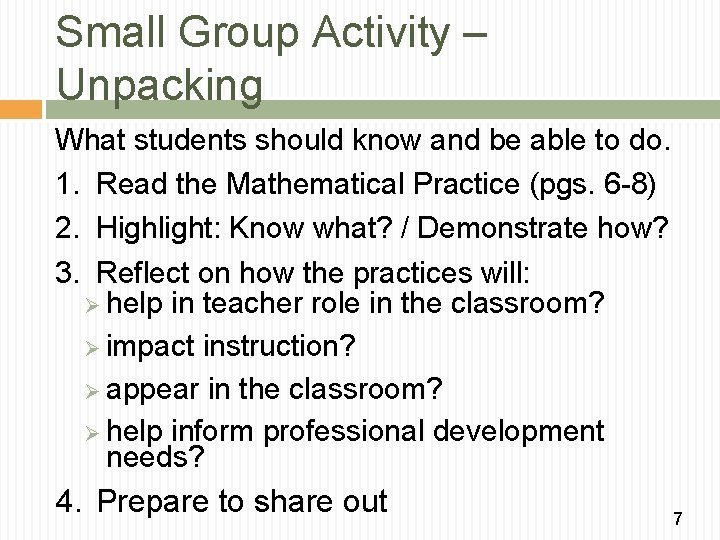Small Group Activity – Unpacking What students should know and be able to do. 1. Read the Mathematical Practice (pgs. 6 -8) 2. Highlight: Know what? / Demonstrate how? 3. Reflect on how the practices will: Ø help in teacher role in the classroom? Ø impact instruction? Ø appear in the classroom? Ø help inform professional development needs? 4. Prepare to share out 7Group Sorting Activity: Connect Practice Standards to Fraction Lesson Materials: �Mathematical Practices Sorting Mat �Teacher Action sorting cards Match the learning activity to the best Mathematical Practice that will be demonstrated by students 8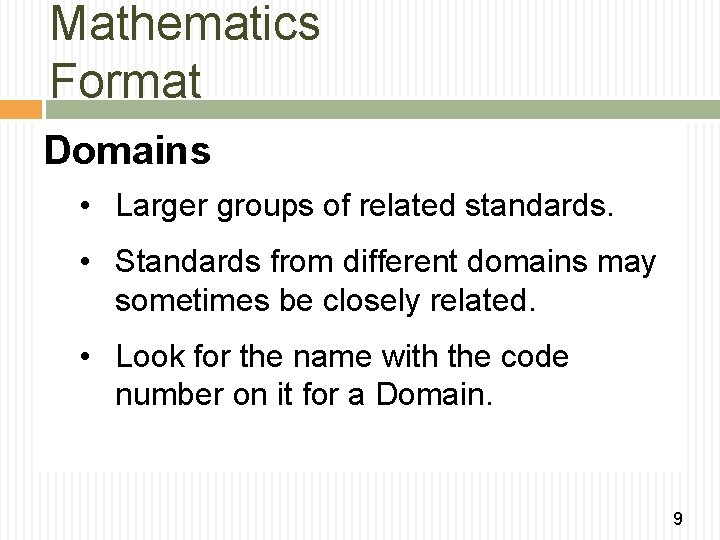Mathematics Format Domains • Larger groups of related standards. • Standards from different domains may sometimes be closely related. • Look for the name with the code number on it for a Domain. 9Mathematics Format Clusters • Groups of related standards. • Standards from different clusters may sometimes be closely related, because mathematics is a connected subject. • Clusters appear inside domains. 10Mathematics Format Standards • Define what students should be able to understand be able to do. • Standards are part of a cluster. 11Common Core Format 12 K-8 Grade Domain Cluster Standards High School Conceptual Category Domain Cluster Standards 12K-8 Grade Level Overview Standard Grade Page K 9 1 13 2 17 3 21 4 27 5 33 6 39 7 46 8 52 Domain Cluster 13Common Core – Domain q Domains are overarching big ideas that connect topics across the grades q Descriptions of the mathematical content to be learned elaborated through clusters and standards 14Common Core – Clusters q May appear in multiple grade levels in the K-8 Common Core. There is increasing development as the grade levels progress q What students should know and be able to do at each grade level q Reflect both mathematical understandings and skills, which are equally important 15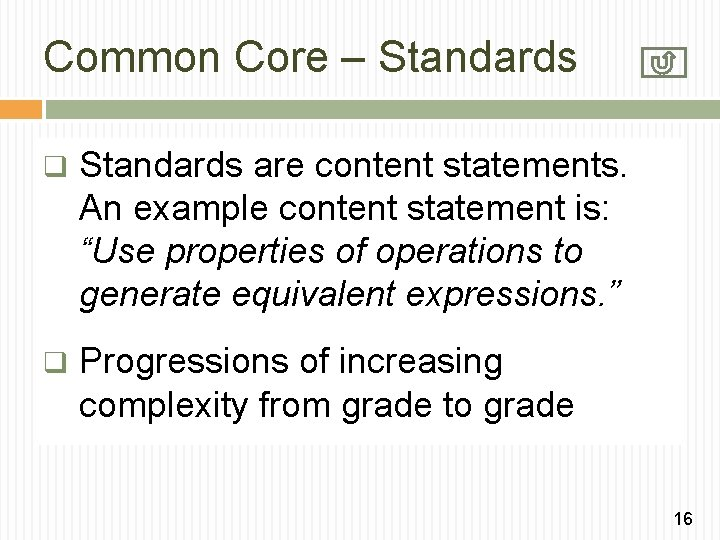Common Core – Standards q Standards are content statements. An example content statement is: “Use properties of operations to generate equivalent expressions. ” q Progressions of increasing complexity from grade to grade 16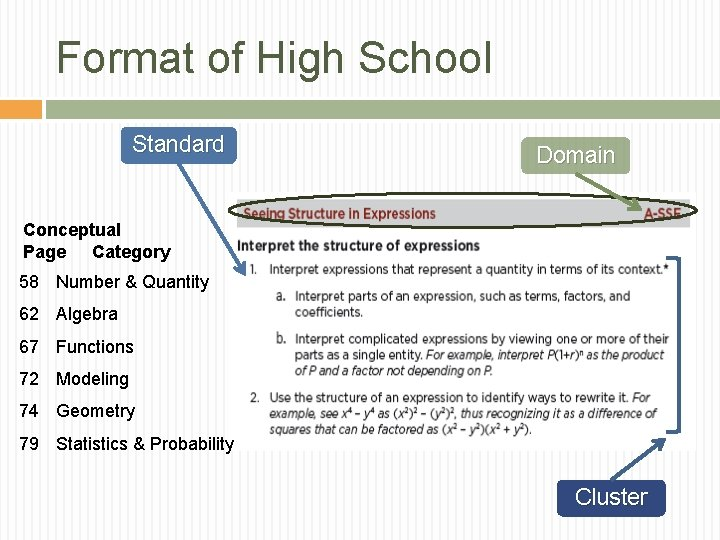Format of High School Standard Domain Conceptual Page Category 58 Number & Quantity 62 Algebra 67 Functions 72 Modeling 74 Geometry 79 Statistics & Probability Cluster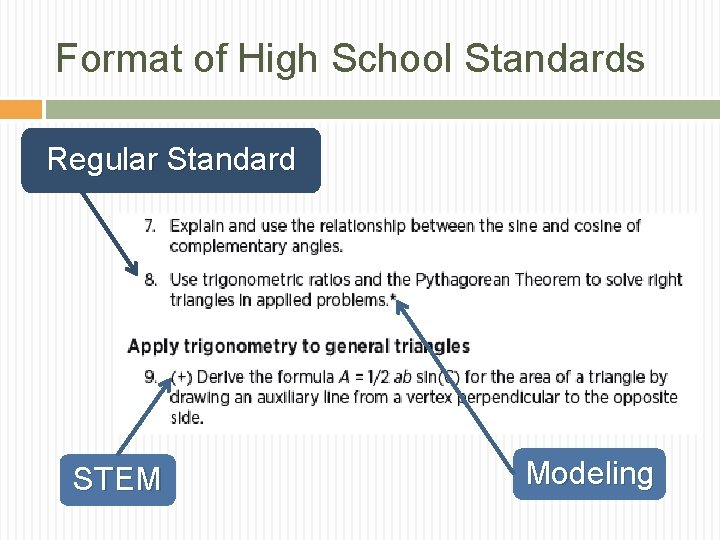Format of High School Standards Regular Standard STEM ModelingDomains K-8 / HS Conceptual Categories 19Coding Key for Math Standards CCMA. K 12. MP. # = Mathematical Practice Grades K-5 Key Grades 6 -8 Key CCMA. K. CC. # = Counting and Cardinality CCMA. K. OA. # = Operations and Algebraic Thinking CCMA. 6. RP. # = Ratios and Proportional Relationships CCMA. 6. NS. # = The Number System CCMA. K. NBT. # = Number and Operations on Base Ten CCMA. 6. EE. # = Expressions and Equations CCMA. K. NF. # = Number and Operations – Fractions CCMA. 6. G. # = Geometry CCMA. K. MD. # = Measurement and Data CCMA. K. G. # = Geometry CCMA. 6. F. # = Functions CCMA. 6. SP. # = Statistics and Probability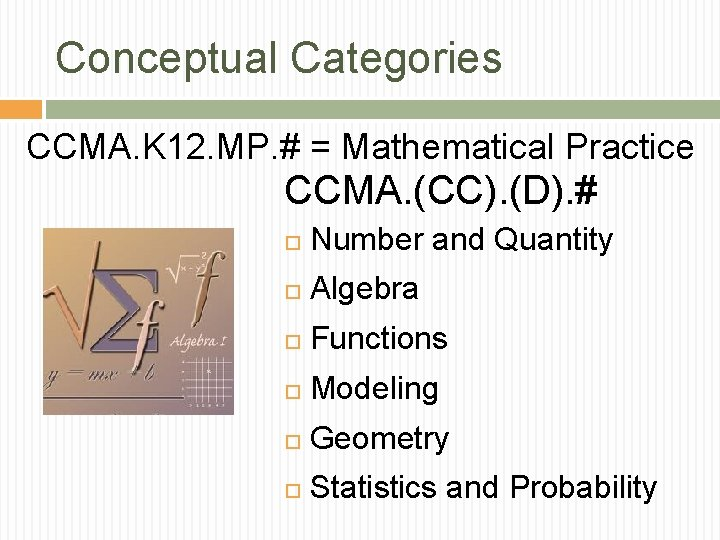Conceptual Categories CCMA. K 12. MP. # = Mathematical Practice CCMA. (CC). (D). # Number and Quantity Algebra Functions Modeling Geometry Statistics and Probability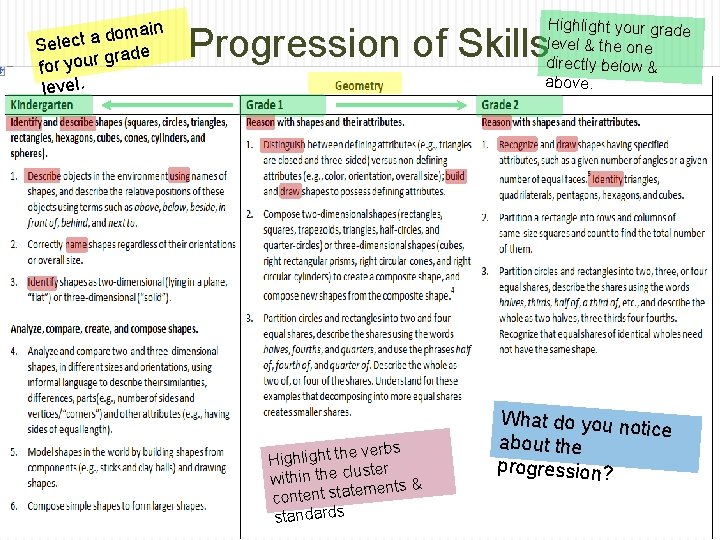main o d a t c Sele grade r u o y r fo level. Highlight your grade level & the one directly below & above. Progression of Skills e verbs Highlight th luster within the c ments & te ta s t n te n o c standards What do you no tice about the progression?Small Group Activity – Unpacking Find your Grade Level in the CCSS Document Look through the Domains & Standards Complete the Unpacking handout; identify concepts that are: o o New The Same Not Included Located in another grade level 23Article Reading Read the article and highlight two sentences and two words that you think is particularly important. First Round: (1 minute each person) Each person shares one sentence and why significant. Identify location with group – page/paragraph – read sentence out loud; share reason selected Second Round: (1 minute each person) Each person shares the word and why significant. Third Round: (10 minutes total) 24Connecting Mathematical Practices w/ Content Standards Small Group Activity pg. 1 � Find the first page for your grade level, locate the instructional focus “critical areas”, and list the key concepts for each area. � Follow the next set of directions Small Group Activity pg. 2 � Follow directions for the activity. � You find a different standard for each mathematical practice 25Rubric Level Evaluatio 4. 0 n Level 3. 0 Target Level 2. 0 Level 1. 0 Level 0. 0 In addition to score 3. 0, teacher can explain how the Mathematics Practices and Mathematics Content Standards interconnect with each other and why both are necessary for Mathematics instruction. The teacher can explain the design and organization of the MCCS and can integrate the Mathematics Practices and Content Standards when developing unit goals. Teacher can explain the how the MCCS is organized but needs assistance in integrating the Mathematical Practices and Concept Standards when developing unit goals. With help, teacher can explain the design of the MCCS and can integrate the Mathematical Practices and Concept Standards when developing unit learning goals. Even with help, no understanding of the Mathematics CCS or the Mathematical Practices and Mathematic Content Standards is demonstrated. 26Rubric /Lesson Plan Course Name Mathematics / Grade 1 Standard(s): MACC. 1. OA. 1 Use addition and subtraction within 20 to solve word problems involving situations of adding to, taking from, putting together, taking apart, and comparing, with unknowns in all positions, e. g. , by using objects, drawings, and equations with a symbol for the unknown number to represent the problem. Note: See Table 1 The student will understand and/or be able to solve addition and subtraction problems within 20 that involve comparing, with unknowns in all positions, using models, explanation, and equations. Learning Goal(s)/ Objective(s) Essential Question(s) Rubric/Evaluatio n How can you solve addition and subtraction problems that involve comparing with unknowns in all positions? Level In addition to Score 3. 0, in-depth inferences and applications that go beyond what was 4. 0 taught the student is able to: Create and solve their own original addition and subtraction problems within 20 that involve comparing, with unknowns in all positions, using models, explanation, and equations. Level The student understands and is able to: 3. 0 Solve addition and subtraction problems within 20 that involve comparing, with unknowns in all Target positions, using models, explanation, and equations. The student exhibits no major errors or omissions Level With help, a partial understanding of some of the simpler details and processes as the 2. 0 student is able to: Solve addition and subtraction problems within 20 that involve comparing, with unknowns in two of three positions, using two or three strategies included in the Score 3. 0 target. However, the student exhibits major errors or omissions regarding the more complex ideas and processes. Level With help, a partial understanding of some of the simpler details and processes as the 1. 0 student is able to: Solve addition and subtraction problems within 20 that involve comparing, with unknowns in one 27 of three positions, using models.Regularly encourage students to demonstrate and deepen their understanding of numbers and operations by solving interesting, contextualized problems and by discussing the representations and strategies they use. 28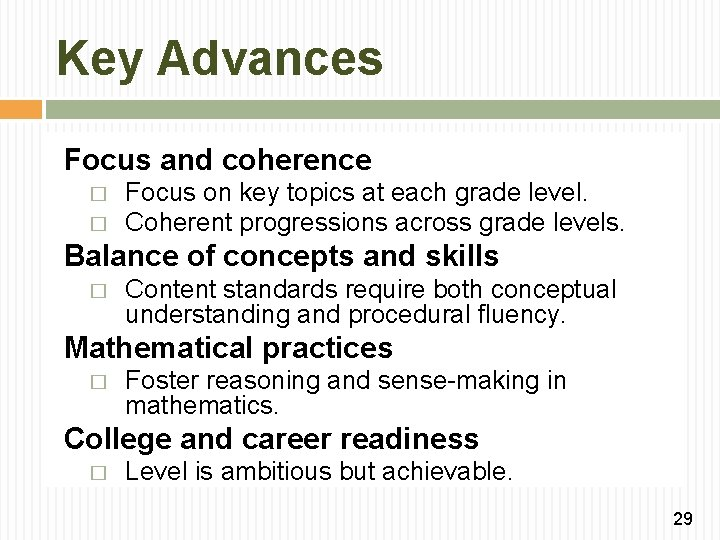Key Advances Focus and coherence � � Focus on key topics at each grade level. Coherent progressions across grade levels. Balance of concepts and skills � Content standards require both conceptual understanding and procedural fluency. Mathematical practices � Foster reasoning and sense-making in mathematics. College and career readiness � Level is ambitious but achievable. 29Mathematical Proficiency “WHERE” THE MATHEMATICS WORK Problem Solving Computational & Procedural Skills DOING MATH Conceptual Understanding “HOW” THE MATHEMATICS WORK “WHY” THE MATHEMATICS WORK 30